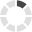## Change CountryLoading... Please wait...

#### Connect with us# Workplace Mathematics: Whole Numbers

## Product Description

Workplace Mathematics: Whole Numbers is part one of the Workplace Mathematics four-part training series.

Course objectives include:

• Learn to recognize and use symbols of arithmetic
• Learn the place value of numbers
• Learn to add whole numbers
• Learn to subtract whole numbers
• Learn to solve arithmetic problems
• Learn to multiply whole numbers
• Learn to divide whole numbers.

The Language of Numbers

• Decimal System
• Symbols and Formats for Representation
• Types of Numbers

• Subtraction
• Solving Arithmetic Problems

Multiplying and Dividing Whole Numbers

• Multiplication
• Division

This series workbook reinforces the purpose and objectives of the accompanying Workplace Mathematics training series.

Workbooks contain lesson introductions, a practice and application area, and lesson progress checks to measure students' learning.

Workplace Mathematics: Whole Numbers is part one of the Workplace Mathemetics three-part training series on DVO.

Course objectives include:

• Learn to recognize and use symbols of arithmetic
• Learn the place value of numbers
• Learn to add whole numbers
• Learn to subtract whole numbers
• Learn to solve arithmetic problems
• Learn to multiply whole numbers
• Learn to divide whole numbers.

Our DVDs are portable and easy-to-use. Training content is "chapterized," or organized by learning objectives to:

• Enable training administrators to cover specific training points and conduct refresher training
• Facilitate classroom discussion.
Quantity:
Length:
SKU: MAT001

## Other Details

RUNTIME:
20
STRINTENG:
MAT001-INT-ENG/IS/